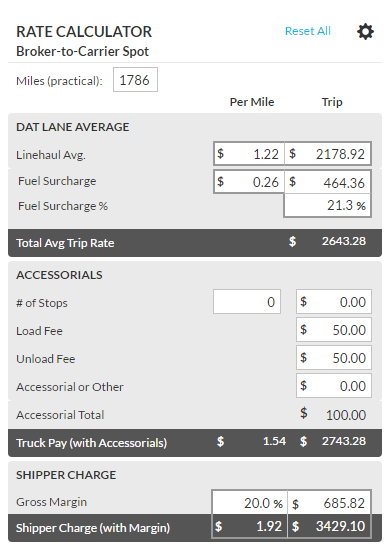The rate calculator customizes the market rate to a rate specific for your load. The rate calculator has fields to enter actual mileage for your trip, any stops, your stop charge (which can be saved) and other surcharges. Adjust the rate to the truck, fuel surcharge and any other values to get to what you expect to pay the truck. Then add on the margin you need right in the calculator to get the amount to charge the shipper. The calculator will auto fill with the current lane you have requested through the single lane request.### DAT Lane Average

The fields listed in this top portion will calculate the amount that you will pay the carrier. The linehaul, mileage and Fuel surcharge are editable fields. The information that is populated in these fields is taken from the lane that is submitted under the single lane request. If a value is changed in any of these fields, the calculator will adjust with the new calculations.### Accessorials

If you are paying the carrier for accessorials, you can adjust the calculator to account for the extra amount that is paid out to the carrier. When you add in a value the amount paid to the carrier/truck is adjusted for you and displays in the “Truck Pay (with Accessorials).”

Clicking on the gearin the upper-right corner of the screen will allow you to set the default fees for accessorials. You can either set those defaults or enter in the values when calculating what you are paying to the carrier.### Gross Margin

The gross margin will calculate your profit margin. Type in the percentage that you want to add to the rate and the calculator will display how much you will make and how much you need to quote to your shipper.

Gross Margin Calculation

• Gross Margin % Fixed - Formula to calculate the Gross Margin Total is:
Gross Margin Total = Gross Margin % * Pay to Truck / (1 – Gross Margin %)
Example: The 2 known variables are Gross Margin % and Pay to Truck. This example is going to use 20% (0.2) and 100.

Gross Margin % = 20% (0.2)
Pay to Truck = 100
1 = Whole Shipper Rate (100%)
Gross Margin Total = X

Formula after plugging in the values: X = (0.2) * 100 / (1 – (0.2))
X = 20 / (0.8)
X = 25

Gross Margin Total using a Gross Margin % of 20%(0.2) and Pay to Truck of 100 equals 25

• Gross Margin Total Fixed - Formula to calculate the Gross Margin % is:
Gross Margin % = Gross Margin Total / (Pay to Truck + Gross Margin Total)
Example: The 2 known variables are Gross Margin Total and Pay to Truck. This example is going to use 25 and 100.

Gross Margin Total = 25
Pay to Truck = 100
Gross Margin % = X

Formula after plugging in the values: X = 25 / (100 + 25)
X = 25 / 125
X = 20% (0.2)

Gross Margin % Using a Gross Margin Total of 125 and Pay to Truck of 100 equals 20% (0.2).